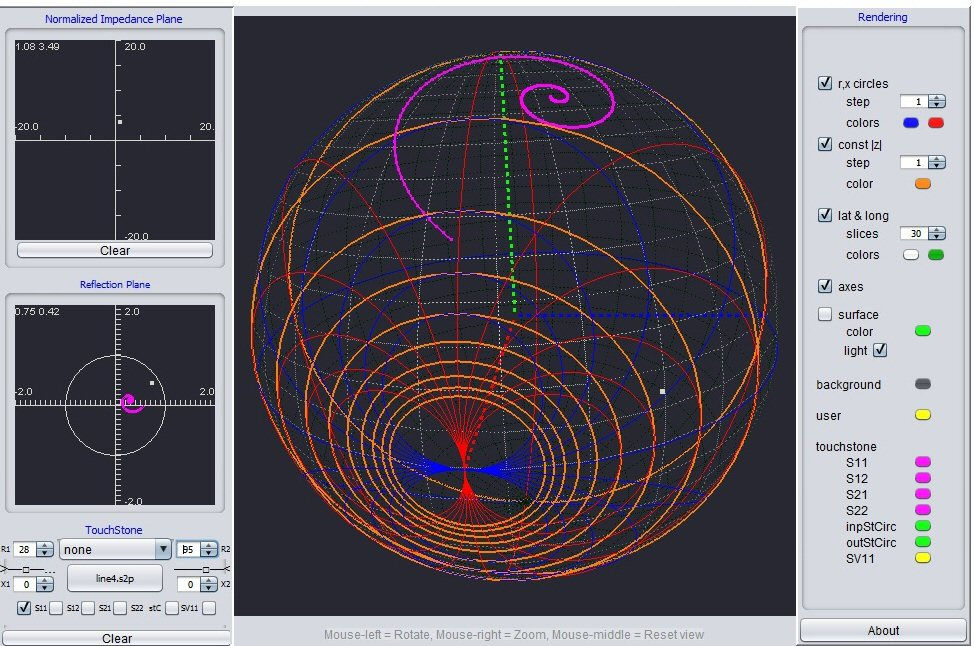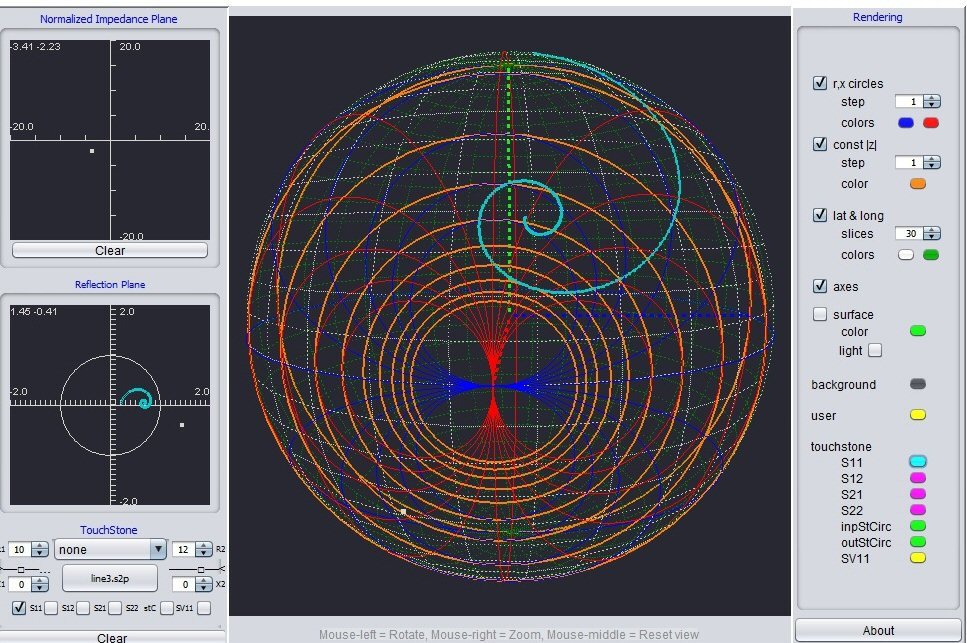# Three Dimensional Smith Chart

Our friends over at Keysight hosted a full hour-long webcast on how to use Python to boost simulation data processing.  As part of that video, engineer Matt Ozalas talks about how to visualize and understand simulation data. In the short 16-minute clip below, Matt shows an example of how to design a broadband amplifier with a 3D Smith Chart.

Introduction

To have a finite and practical size, the Smith Chart is constrained to the unit circle. Hence, loads with reflection coefficient’s magnitude greater than 1 cannot be plotted. These loads often appear in active circuits and in lossy transmission lines with complex characteristic impedances. The reason for seeking an extension is determined by the desire to have a chart suitable for “including the negative impedances” and lossy transmission lines without sacrificing the usual benefits the Smith chart usually offers.

Starting in 2007 many attempts where done in order to overcome the limitation of the classical Smith chart. The spherical approaches presented between 2007-2010 in known journals (MW Magazine or MOPTL) failed to unify the active and passive circuit design in an unitary manner since they were based on empirical methods or arithmetical manipulation.

The 3D Smith chart presented here is based on the novel pure mathematical-practical model proposed in “IEEE Microwave and Wireless and Components Letters” June 2011 article “A 3D Smith chart based on the Riemann for Active and Passive Microwave Circuits”. See references below.

The images plotted are based on the 3D Smith chart Java tool developed by the first author of the IEEE MWCL June 2011 paper Andrei Muller [www.3dsmithchart.com]3D Smith chart theory

The mathematical trick proposed by the 3D Smith chart article is to extend the reflection coefficient’s plane with the point at infinity (thus to accept the division with 0) and work in the extended reflection coefficient’s plane  Ĉ= (ℂ∪ {∞})).

Then a one-to-one mapping between the points of the 2D Smith chart (extended to infinity) in 2D and the inverted Riemann sphere is performed.

The 2D extended Smith chart is considered to be the equatorial plane of the Riemann sphere and then after the mapping one gets the 3D Smith chart which is on the surface of the unit sphere.

The 3D Smith chart geometry is very simple: it is made by circles on the surface of the unit sphere. The circles that never got together in the 2D chart meet in the South pole. The constant r, constant x lines of the impedance plane are transformed into circles on the surface of the Riemann sphere. The North hemisphere surface embodies all the passive circuits, the South is for circuits with negative resistance, the West hemisphere for capacitive circuits while the East for inductive circuits. The Greenwich meridian is made by pure resistive circuits.

Due to the Riemann sphere properties one can rotate the 3D Smith chart and obtain the entire impedance plane too (thus it is a generalized dual Smith chart too since the constant abs(z) circles of the impedance plane are also mapped into circles on the 3D Smith chart surface).

3D Smith chart usage

The usage is straight-forward, since the analogy with google-earth is possible. One has to get to the North pole to get the perfect matching (the South pole is infinite mismatch). Moving on a latitude=moving on a lossless line (the latitudes are related with the absolute value of the reflection coefficient) (North pole=0 reflection, Equator=1, South pole infinity).

3D Smith chart applications

The main applications of the 3D Smith chart are in amplifier stability, oscillator design, and lossy matching and problems involving the impedance plane (since one can rotate the 3D chart and obtain the impedance directly.

The 3D Smith chart free demo tool on [www.3dsmithchart.com] shows these properties.

Andrei has updated his video explanation (below).

References

Andrei A. Muller, P. Soto, D. Dascalu, D. Neculoiu and V. E. Boria, “A 3D Smith Chart based on the Riemann Sphere for Active and Passive Microwave Circuits,” IEEE Microwave and Wireless Components. Letters, vol 21, issue 6, pp 286-288, june, 2011

The Java 3D Smith chart tool was presented in 10 minutes during European Microwave week 2011 in the following event:

Andrei Muller, EUMW 2011, Microwave Fundamentals, EUMW 2011, 13 October, Manchester

Other papers (2007-2010) that tried to extend in an empirical way or with arithmetical manipulation way the Smith chart are:

C. Zelley, “A spherical representation of the Smith Chart,” IEEE Microwave, vol. 8, pp. 60-66, Jun. 2007.

Y. Wu, Y. Liu, and H. Huang, “Extremely generalized planar Smith Chart based on Möbius transformations,” Microwave Opt. Technol. Lett., vol. 51, pp. 1164-1167, May 2009.

Y. Wu, Y. Zhang, Y. Liu, and H. Huang, “Theory of the spherical generalized Smith Chart,” Microwave Opt. Technol. Lett., vol 51, pp. 95-97, Jan. 2009.

Author : Unknown Editor